# Forces, by Claude Théberge at Square Viger

Howdy!

This is called death by neglect, and it is horrible.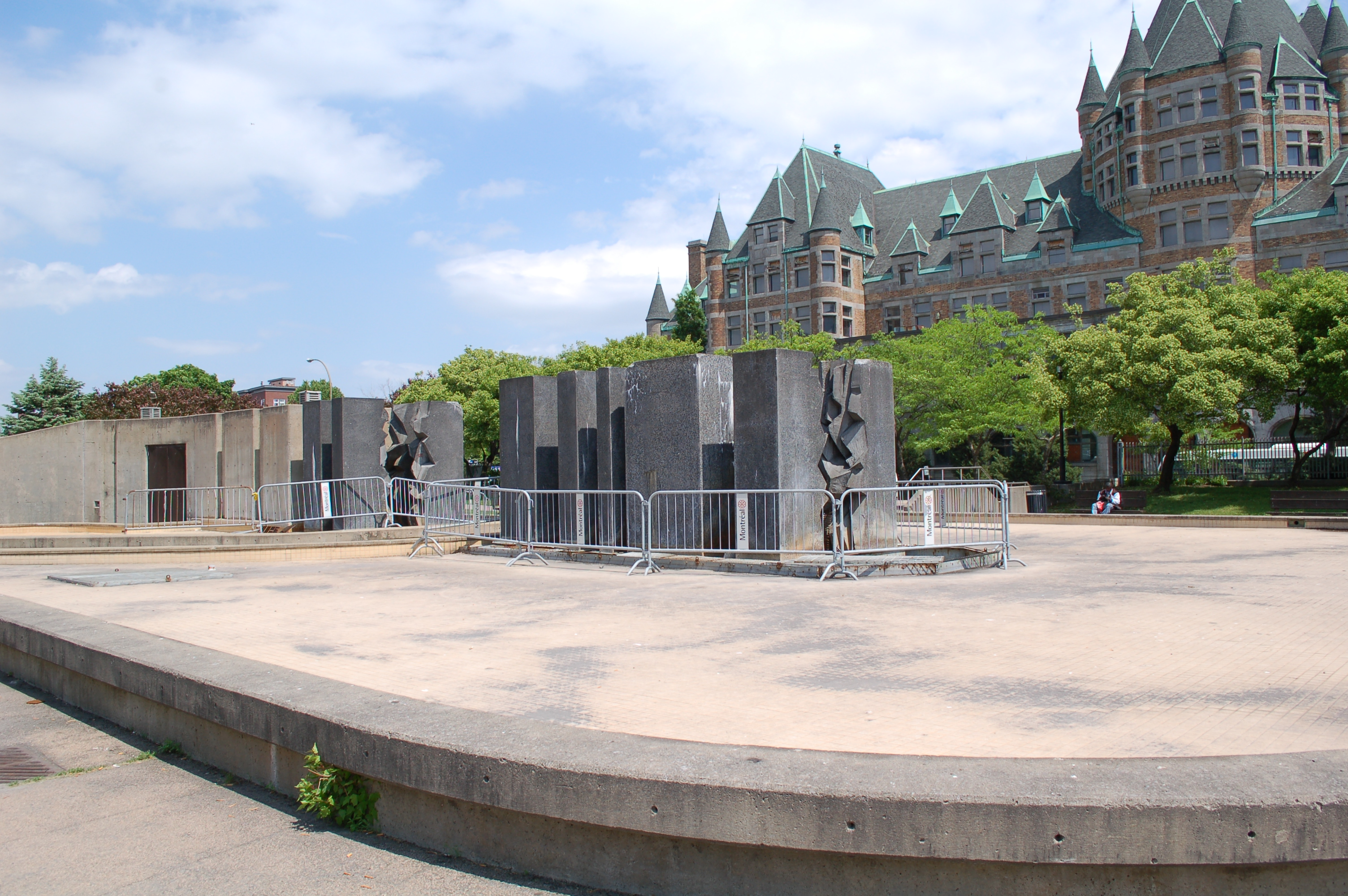Forces, by Claude Théberge at Square Viger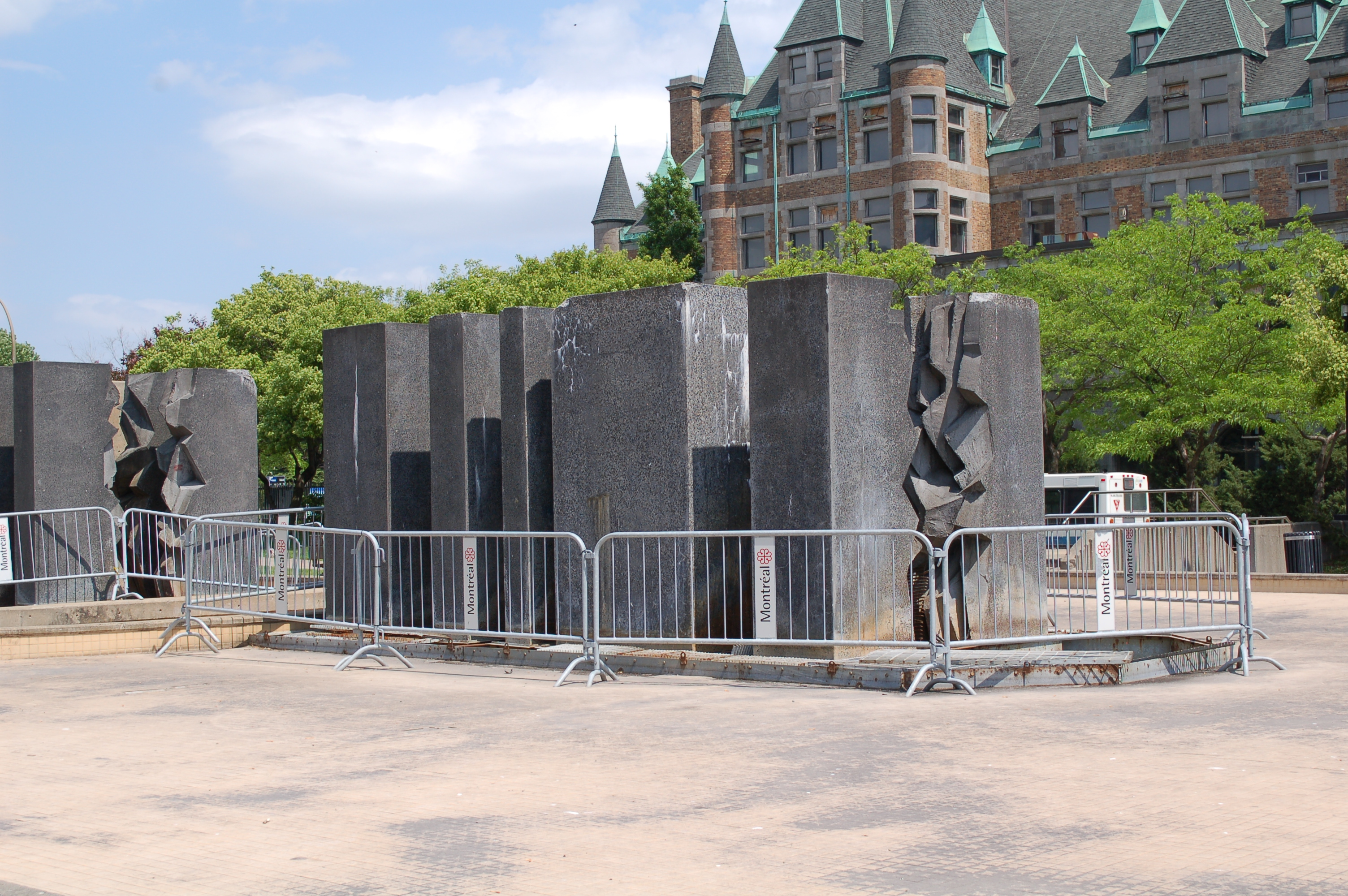Forces, by Claude Théberge at Square Viger

Believe it or not, it was built in 1985.Forces, by Claude Théberge at Square VigerForces, by Claude Théberge at Square VigerForces, by Claude Théberge at Square VigerForces, by Claude Théberge at Square Viger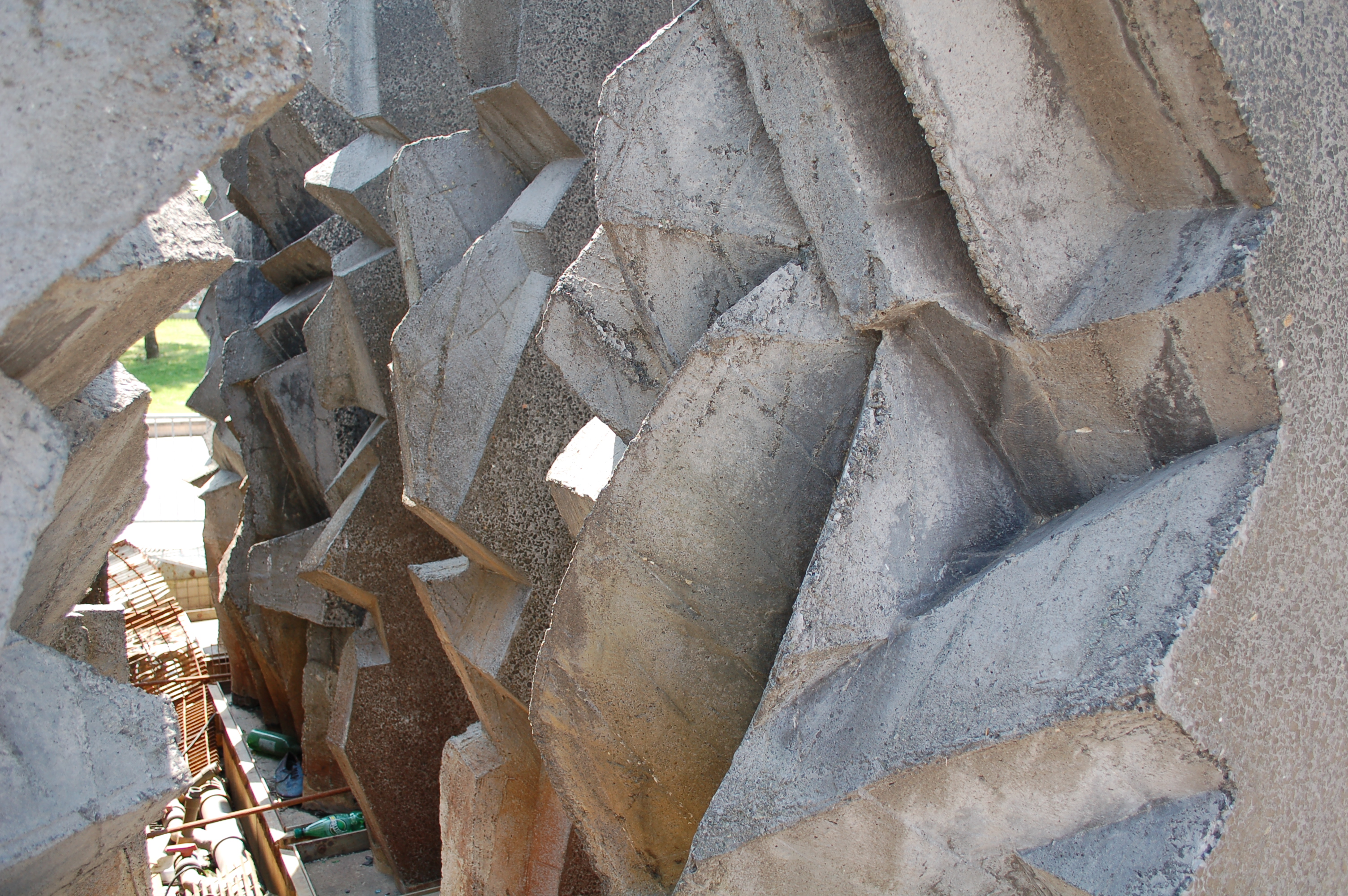Forces, by Claude Théberge at Square Viger

More information about it and him can be found here and here.Forces, by Claude Théberge at Square Viger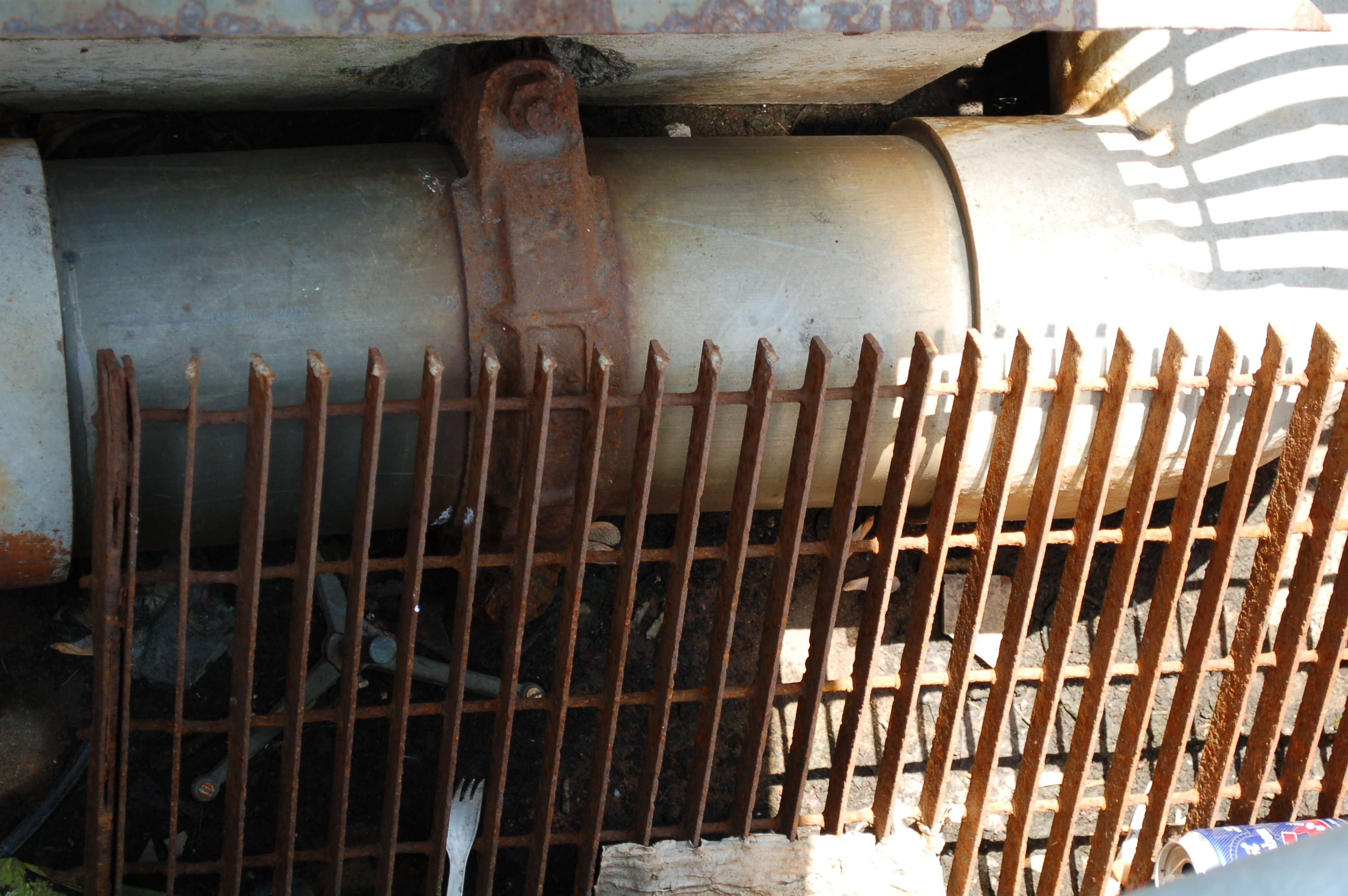Forces, by Claude Théberge at Square VigerForces, by Claude Théberge at Square VigerForces, by Claude Théberge at Square VigerForces, by Claude Théberge at Square Viger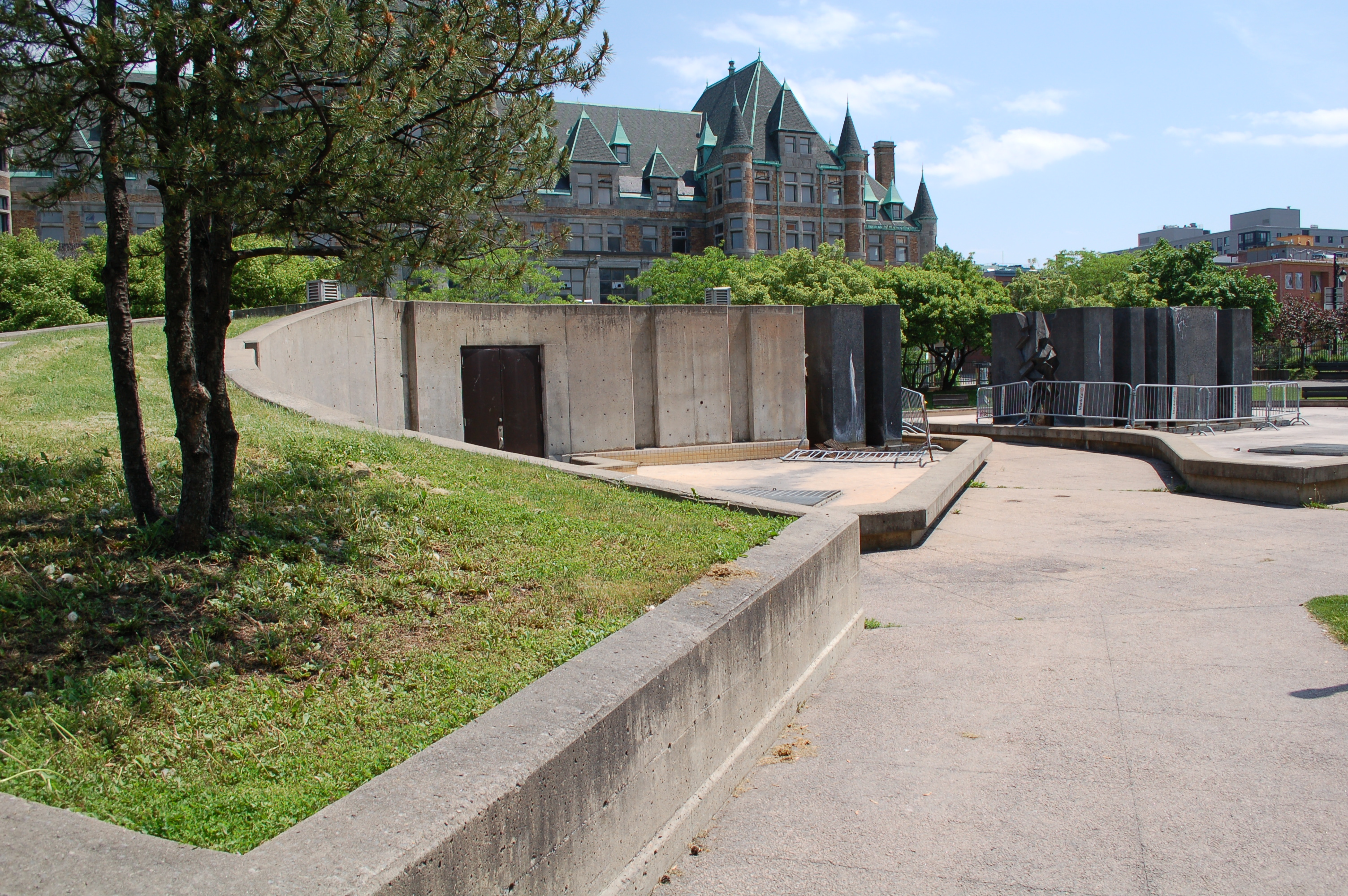Forces, by Claude Théberge at Square Viger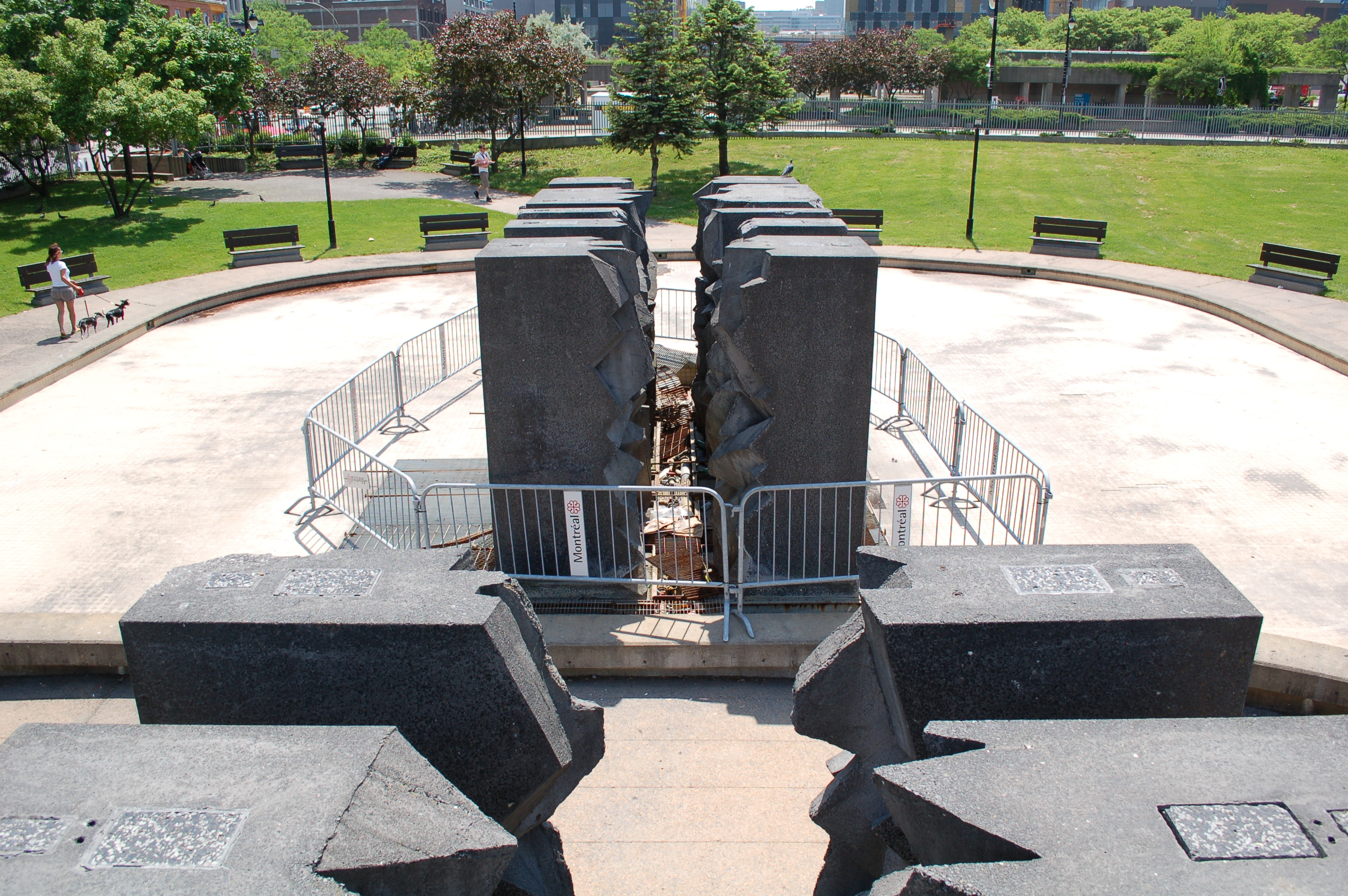Forces, by Claude Théberge at Square Viger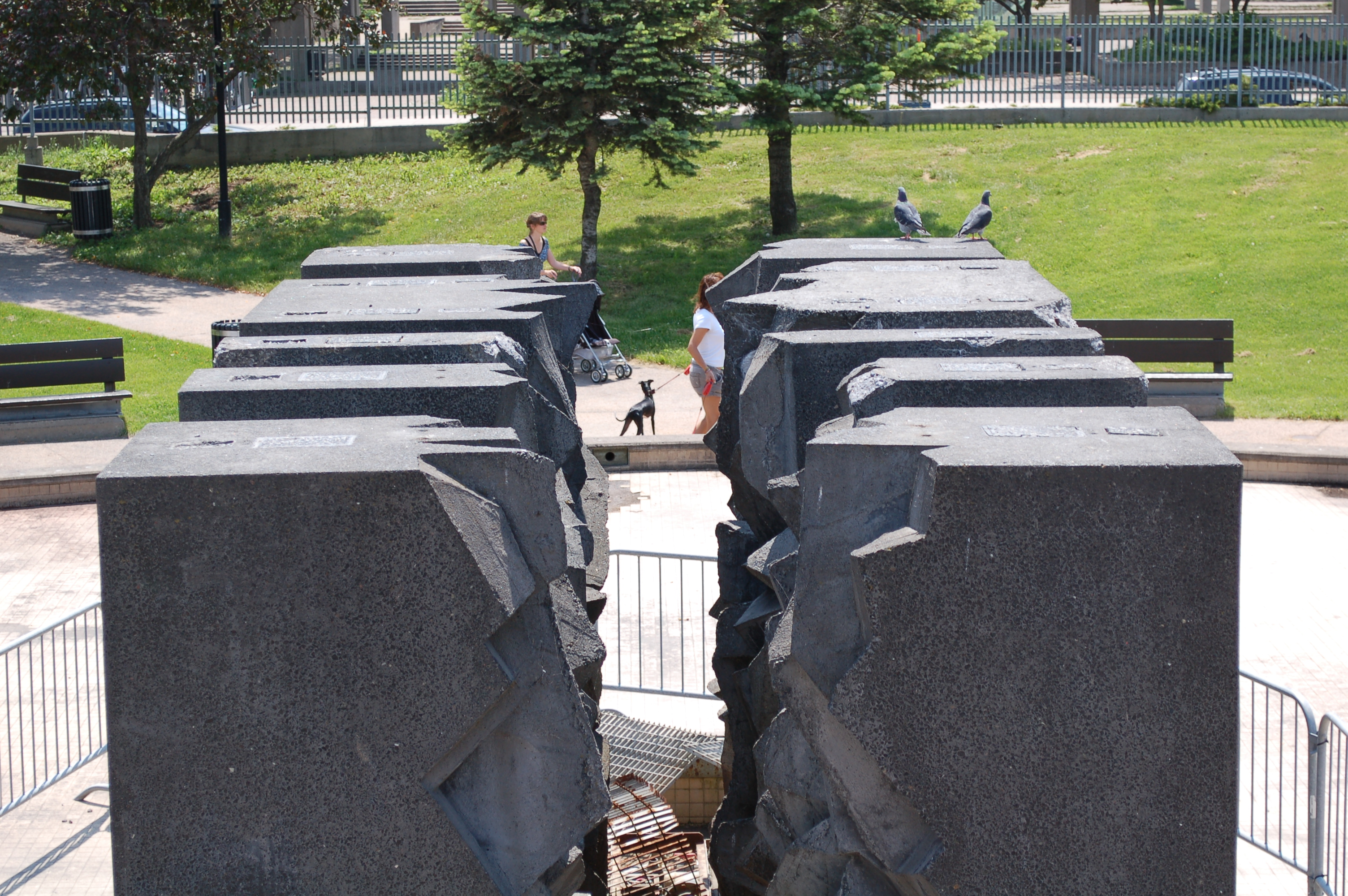Forces, by Claude Théberge at Square VigerThe view from Forces, by Claude Théberge at Square Viger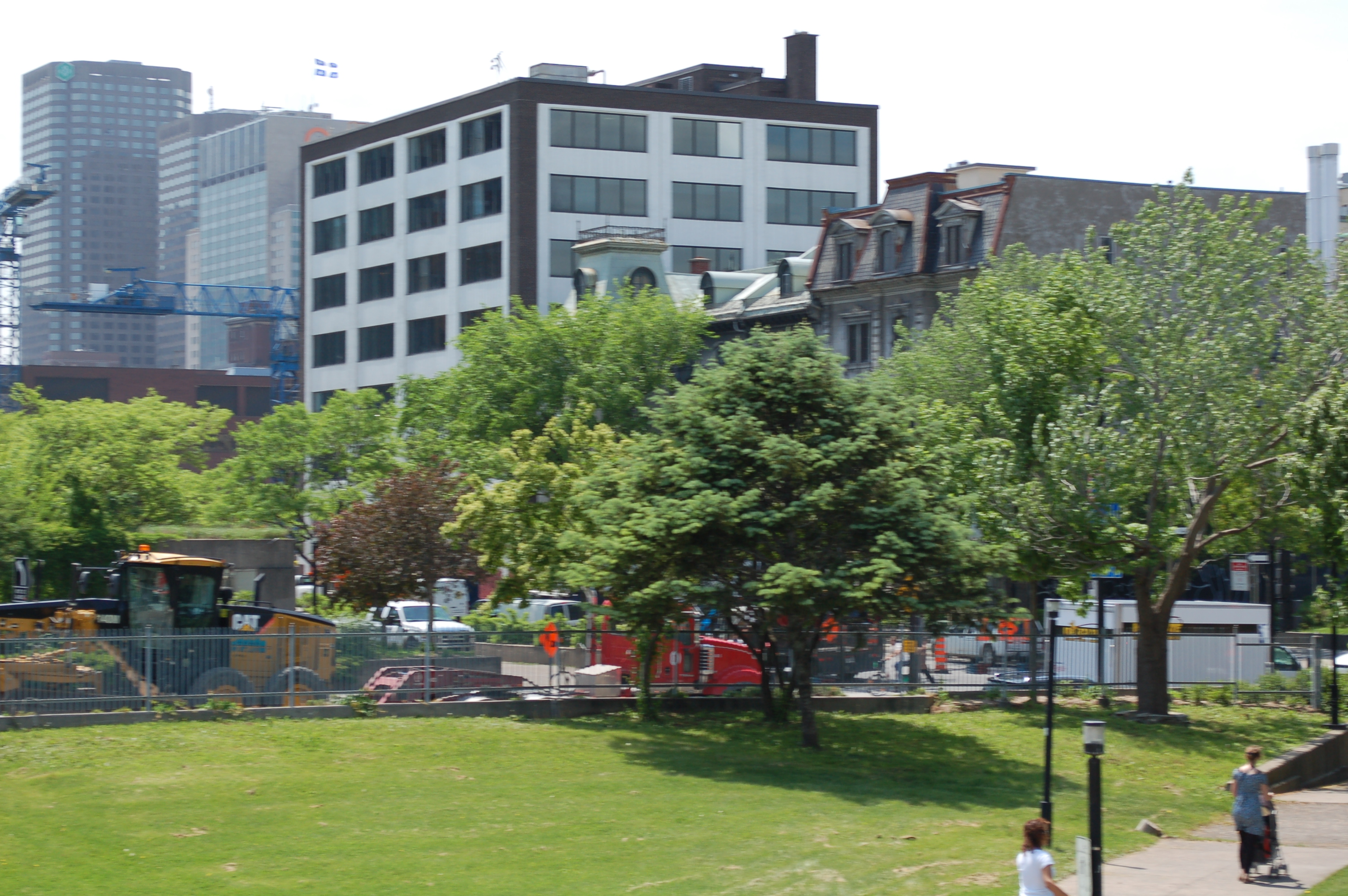The view from Forces, by Claude Théberge at Square VigerThe view from Forces, by Claude Théberge at Square VigerThe view from Forces, by Claude Théberge at Square VigerThe view from Forces, by Claude Théberge at Square VigerThe view from Forces, by Claude Théberge at Square Viger